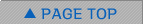Events / Media

Data Assimilation Seminar Series

Mr. Sho Yokota (Feb. 17, 2016, 14:30-)

Affiliation JMA MRI Comparison between LETKF and EnVAR with observation localization Ensemble Kalman filter explicitly calculates the analysis using assumptions of the linear observation operator and the Gaussian probability distribution function of analysis variables. To avoid errors based on these assumptions, ensemble-based variational data assimilation (EnVAR) is one of the useful methods. We added the EnVAR option to the local ensemble transform Kalman filter (LETKF) system. This EnVAR formulation uses observation localization, and differs from the LETKF formulation only in two points: (i) not assuming linearity of the observation operator and (ii) calculating it globally. In this seminar, this EnVAR will be compared to LETKF using single-observation assimilation experiments and the observation system simulation experiments with a low-resolution global model SPEEDY. Particularly because the observation operator is globally calculated in this EnVAR, this EnVAR has an advantage over LETKF. Link to PDF file